﻿ Modeling and Simulation of a New Duty-Cycle Modulation Scheme for Signal Transmission Systems

### Modeling and Simulation of a New Duty-Cycle Modulation Scheme for Signal Transmission Systems

Leandre Nneme Nneme, Jean MbihiOPEN ACCESSPEER-REVIEWED

## Modeling and Simulation of a New Duty-Cycle Modulation Scheme for Signal Transmission Systems

Leandre Nneme Nneme1,, Jean Mbihi1

1Department of Electrical and Electronics Engineering, ENSET (University of Douala), Douala, Cameroon

### Abstract

This paper studies a new signal transmission scheme founded on duty-cycle modulation. The proposed signal transmission system consists of a low cost duty-cycle modulation circuit, a transmission line model and a linear demodulator. The study of the signal transmission system is conducted using analytical developments and virtual simulation models. Then, the results obtained under a variety of modulating waveforms including standard signals and arbitrary waveforms, show the high quality of the proposed duty-cycle modulation scheme for signal transmission systems.

### At a glance: Figures

1234
Prev Next

• Nneme, Leandre Nneme, and Jean Mbihi. "Modeling and Simulation of a New Duty-Cycle Modulation Scheme for Signal Transmission Systems." American Journal of Electrical and Electronic Engineering 2.3 (2014): 82-87.
• Nneme, L. N. , & Mbihi, J. (2014). Modeling and Simulation of a New Duty-Cycle Modulation Scheme for Signal Transmission Systems. American Journal of Electrical and Electronic Engineering, 2(3), 82-87.
• Nneme, Leandre Nneme, and Jean Mbihi. "Modeling and Simulation of a New Duty-Cycle Modulation Scheme for Signal Transmission Systems." American Journal of Electrical and Electronic Engineering 2, no. 3 (2014): 82-87.

 Import into BibTeX Import into EndNote Import into RefMan Import into RefWorks

### 1. Introduction

The duty-cycle modulation (DCM) is a class of signal conversion techniques in which an input signal x is transformed into a periodic switching wave xm(t) with period Tm(x), and duty-cycle Rm(x) = Ton(x)/Tm(x), where Ton(x) is the pulse width of xm(t). Generally speaking, Rm(x) is a monotonous function of x since it should be possible to extract the modulating x from Rm(x) using a simple demodulation circuit if any.

As reported in , although the general DCM principle is not new, over years many types of implementation techniques with specific characteristics have been built for application in a variety of application areas. In the pulse width modulation (PWM) technique where Tm(x) = Tm is kept constant, a basic PWM circuit requires a triangle wave oscillator and a comparator , and a whole minimal implementation requires three integrated operational amplifiers and four passive components. In the sigma-delta modulation (SDM) policy where both Ton(x) and T(x) depend on x, a standard SDM circuit outlined in  consists of a summing amplifier, an integrator, a controlled latch, a feedback mechanism, a separate clock source, and more. Furthermore, a few novel DCM configurations are encountered in the space of modern patents ([4, 5]). In the patent , the novel DCM circuit presented structurally behaves as a closed loop system, consisting of a controlled Schmitt trigger and a summing integrator, in which case a whole realization requires at least two integrated circuits and seven passive elements. Whereas in the patent , the new modulation circuit presented consists of a number of building blocks organized according to a feedback configuration, including a summing amplifier, an accumulator, a binary comparator, a delay circuit, a ROM device and more.

Surprisingly, the hardware architecture of existing DCM schemes are notoriously complicated. Unfortunately, according to our best knowledge, a clear proof of the significant profit arising from the structural complexity of the modulation circuit at the expense of its simplicity, appears to be missing in the DCM literature.

Thus, the active need of DCM schemes providing both structural simplicity and high quality, has motivated the development of a class of low cost DCM circuits presented in , consisting of the use of a single integrated operational circuit and a few passive components. Following , further research works have successfully developed low cost DCM schemes for a variety of application areas in industrial electronics ([7, 8, 9]). In , a novel analog-to-digital conversion (ADC) technique is designed and well tested, and its multichannel model is also implemented in . In , a novel digital-to-analog conversion (DAC) technique using low cost duty-cycle modulation, is designed and successfully implemented. Furthermore, it is shown in  that a duty-cycle modulation (DCM) driver for a buck power converter offers best characteristics compared to that of a traditional pulse width modulation (PWM). However, the literature review related to the application of low cost DCM schemes presented above, is mainly limited to instrumentation and industrial electronics engineering.

The new application area of the low cost DCM scheme studied in this paper, is a signal transmission system. The architecture and analytical models of the transmission system are presented in depth in Section 2. Then, Matlab/Simulink model and the simulated results obtained when simulating a prototyping transmission system, are presented in Section 3, whereas the conclusion of the paper is outlined in Section 4.

### 2. Architecture and Analytical Models of DCM-Based Signal Transmission System

The architecture of the proposed DCM-based signal transmission system is presented in Figure1, where its main subsystems are numbered from 1 to 4. It consists of a modulating signal source (N°1), a simple DCM circuit (N°2), a transmission line (N°3) and a demodulation filter (N°4).

The modulating signal source (N°1) might be any testing instrument with a variety of selectable output signals including standard waveforms and white noises.

The simple DCM circuit (N°2) behaves as a controlled oscillator with embedded clock. For a modulating x(t), the Fourier series of the resulting periodic modulated wave could be computed at a given time t as follows :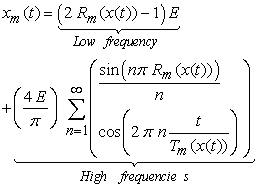(1)

where Rm(x) could be described in its linear range by the following relation ship :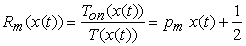(2)

with,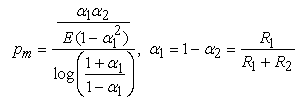(3)

Thus, Equation (1) given (2) shows that the modulating signal x(t) could be recovered from (1) using a suitable low-pass filter with static gain Kf defined as follows: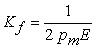(4)

Unfortunately, in the DCM-based signal transmission system considered in this paper, there is a long transmission line between the remote DCM source and the demodulation process, might have significant impacts on the quality of the overall DCM scheme.

The parameters of the transmission line (N°3) between the DCM source and the demodulation filter are defined as follows: d (length), L (inductance per unit length) and C (capacitance per unit length). Assuming that the line is lossless, then the signal xm(z, t) propagating along the line at distance z from the origin z = 0 could be as the following Telegraph’s Equations  :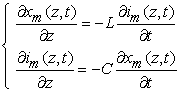(5)

or equivalently,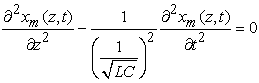(6)

Then, the general solution of Equation (6) at the end of the line where z = d is given as follows :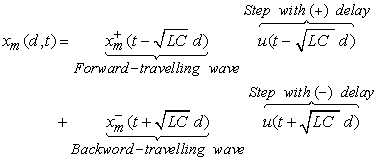(7)

As a conclusion at time t, the modulated 1-bit signal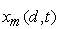available for filtering at the end of the lossless transmission line with length d, behaves as a delayed image of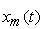=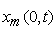.

The demodulation unit (N°3) is a standard second order active filter, with transfer function given as follows :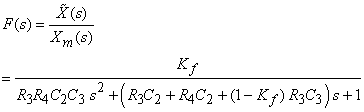(8)

where Kf = 1+ R6/R5 (static gain). As an implication, from Equation (4, the following constraint should be satisfied :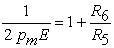(9)

For the sake of simplicity, it is easy to show that Equation (8) could be written as follows: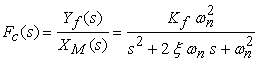(10)

In which case, ωn is the natural frequency (in rad/s), and ξ being the damping coefficient. In addition, for a given cut off frequency fc (in Hz), the related natural frequency could be computed using the following formula :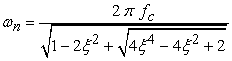(11)

Equations (1)-(11) stands for a complete set of analytical characteristics of the proposed DCM-based signal transmission system.

### 3. Matlab/Simulink Model and Simulation Results of the DCM Transmission System

In engineering, the use of Matlab/Simulink as a suitable framework for rapid simulation of dynamic systems ([14, 15, 16]). Following this common practice, the complete Matlab/Simulink model of the DCM-based transmission system is presented in Figure 2, where the subsystems are numbered according to the same order used in the general DCM architecture shown earlier in Figure 1.

The remote modulating subsystem (N°1) consists of a low frequency virtual instrument with selectable standard waveforms (sine, square, saw teeth) and a chirp signal generator. The readers interested to signal generation algorithms for standard signals and arbitrary waveforms including wavelets, can find more details in ([17, 18, 19]). The selection of signals is conducted using a couple of bus create/select objects. Then, the DCM subsystem is built using the Kirchhoff law applied to the behavior of the modulation circuit observed on Figure 1. These equations are given as follows: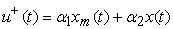(12)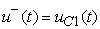(13)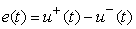(14)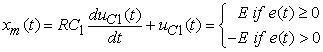(15)

From (4), it is obvious to write an equivalent integral,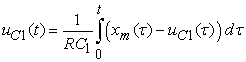(16)

As an implication, a set of nine basic operators available in Simulink toolbox are sufficient for building a complete model of the proposed DCM process.

The lossless transfer line (N°3) is available in Simulink toolbox, and the critical parameter to be specified by the user, is the propagation time delay along the transmission line. It corresponds in Equation (14) to the factor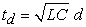. Finally, the model of the demodulation low-pass filter has been implemented with ease using the transfer function objet available in Simulink tool box.

3.2. Simulation Results

The main data used for numerical simulation of the prototyping transmission, is summarized in Table 1.

#### Table 1. Summary of data used for the simulationDownload asPowerPoint Slide

Veiw figureView current table in a new window

For the sake of clarity, the simulation results presented in this Section are organized into set of m coupled figures (Figure m, Figure m+1) for m = 2, 3, …, 9. In each case, Figure m reports the behavior of the DCM scheme assuming that the transmission line is ideal, whereas Figure m+1 stands for the case where the transmission line is assumed lossless under the same modulating input.

As shown from (Figure 5, Figure 6) to (Figure 9, Figure 10) related to the transmission of a variety of waveforms including an arbitrary chirp wave, it is important to observe that, the transmission result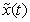obtained under the assumption of ideal transmission line, is almost perfect since the overall outputappears to be an exact copy of the modulating input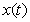. Furthermore, in all cases where the dynamic model of the lossless transmission line is taken into account, the resulting waveobtained is quite closed to. However, a narrow gap observed between the two, is due to the fact that Simulink model of a lossless transmission line is computed from a given time delay Td using a [n/m] Padé approximant . As an implication, the model of the lossless transmission line behaves as an additional rational transfer function (characterized by m zeros and n poles), placed in series upstream the demodulation filter. These findings emerging from the simulation results lead to conclusion of this paper.

Figure 10. Transmission of a chirp signal (Lossless transmission line)

### 4. Conclusion

The analytical developments and the related simulation results presented in this paper, shows the high quality of the proposed DCM scheme for signal transmission systems. Although the simulation has been conducted for low frequency modulating waves, a higher modulating frequency bandwidth might be covered for an optimal choice of the design parameters associated with both the duty-cycle modulator and the low-pass filter. In addition, it would be interesting to study the behavior of the proposed DCM scheme under a more realistic transmission line with losses. Furthermore, it would be also a challenge to build a real prototyping DCM system, in order to show the realistic nature of the proposed signal transmission scheme. These unsolved problems appear to be good opportunities for future research works.

### References

  Roza, E. "Analog-to-digital conversion via duty-cycle modulation", IEEE transactions on circuits and systems II: Analog and digital signal processing, 44 (11) (1997), pp. 907-914.In article  Millman, I., Grabel, A. Micro Electro,ics, 2nd Edition, 10001 pages, 1987, © Mc Graw Hill.In article  Sangil Park, Motorola digital signal processors – Principles of sigma-delta modulation for analog-digital (February, 2010), http://digitalsignallabs.com/SigmaDelta.pdf, pp 1.1-9.6.In article  Harvey, P., C. Transmitting a signal using duty-cycle modulation, United State Patent N° 6 111 680, August 29, 2000.In article  Takamuki, H. Sigma-Delta modulation circuit. United State Patent N° 6 057 794, May 2, 2000.In article  Mbihi, J., Ndjali Beng, F., Mbouenda, M., Modeling and simulation of a class of duty-cycle modulators for industrial instrumentation, Iranian Journal of Electrical and Computer engineering, 4 (2)2 (2005) pp. 121-128In article  Mbihi, J., Ndjali Beng, F., Kom, M., and Nneme Nneme, L. A novel analog-to-digital conversion technique using nonlinear duty-cycle modulation, International Journal of electronics and computer science engineering, 1(3), 2012, pp. 818-825.In article  Mbihi, J., Nneme Nneme, L. A multichannel analog-to-digital conversion technique using parallel duty-cycle modulation, 1(3), 2012, pp. 826-833.In article  Moffo Lonla, B., Mbihi, J., Nneme Nneme, L. and Kom, M. A novel digital-to-analog conversion technique using Duty-cycle modulation, International Journal of circuits, systems and signal processing, 1(7) 2013, pp. 47-49.In article  Mbihi, J., Nneme Nneme, L. A novel control scheme for buck power converters using duty-cycle modulation, International Journal of power electronics, 5(3), 2013, pp. 185-199.In article  Nitsch, J., Tkachenko, S. Telegrapher equations for arbitrary frequencies and modes: Radiation of an infinite, lossless transmission line, Radio Science, 30(2), 2004.In article  Engineering Electromagnetics, 4th edition by William Hayt, 1981. Chapter 12, section 1, "The Transmission Line Equations".In article  Ogata, K. "Modern control engineering – Second Edition", pp 526-528, prentice hall, 1990.In article  Tilak Thakur, and Kumar Gupta S., Modeling of a Stand-Alone Induction Generator on Load Using MATLAB Simulink, Journal of Emerging Trends in Engineering and Applied Sciences, Vol. 3, No. 4, 2012, pp. 729-733.In article  A. Aldair A. A., Hardware Implementation of the Neural Network Predictive Controller for Coupled Tank System, American Journal of Electrical and Electronic Engineering. 2014, 2(2), pp. 40-47.In article  Karthikeyan, V., Vijayalakshmi, V.J. and Jeyakumar P., Selective Harmonic Elimination (SHE) for 3-Phase Voltage Source Inverter (VSI), American Journal of Electrical and Electronic Engineering. 2014, 2(1), pp. 17-20.In article CrossRef  Hung-Chen, C. Square/Triangular wave generator using Single DVCC and three grounded passive components, American Journal of Electrical and Electronic Engineering, 1(2) 2013, pp. 32-36.In article CrossRef  Mbihi, J. and Motto, A. Instrumentation virtuelle assistée par ordinateur - Principes et techniques – Cours et exercice, 240 p., Ocober 2012, © Ellipse, Paris.In article  J. Mbihi, J., Tene, N. and Takoufet, K., Etude et prototypage d’un générateur de signaux arbitraires à vocation didactique, Journal sur l'Enseignement des Sciences et des Technologies de l'Information et des Systèmes, Vol 9, N°2, 2010, EDP SciencesIn article  Baker, G. A., and Graves-Morris, P., Padé Approximants, second Edition, Encyclopedia of Mathematics and its Applications, March 11, 2010.In article